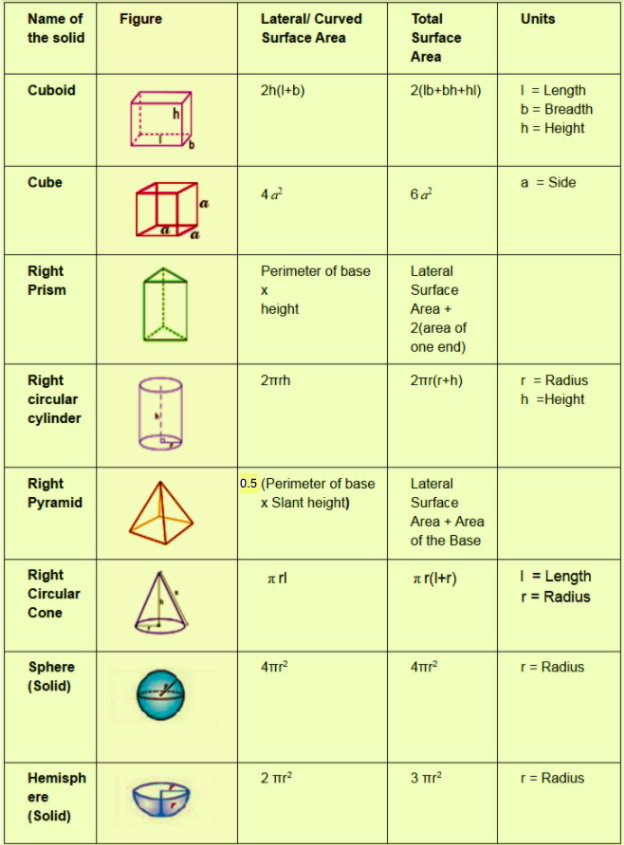# Surface Area Formulas

Surface area formulas in geometry refer to the lateral surface and total surface areas of different geometrical objects. To recall, the surface area of an object is the total area of the outside surfaces of the three-dimensional object i.e, the total sum of the area of the faces of the object. It is measured in terms of square units. In other words, the surface area is the sum of all the areas of all the shapes that cover the surface of the object. On the other hand, the lateral surface area refers to the area of the sides of a shape, excluding its base and top area.

Try Various Surface Area Calculators Here: Maths Calculators

Here in this page, you can see a list of Surface Area Formulas for all kinds of available geometrical figures.

## Surface Area Formulas:

Shape Lateral Surface Area (LSA) Total Surface Area (TSA)
Cuboid 2h(l + b) 2(lb + bh + lh)
Cube 4a2 6a2
Right Prism Base perimeter × Height LSA + 2 (area of one end)
Right Circular Cylinder 2πrh 2πr(r + h)
Right Pyramid (1/2) Perimeter of base × Slant Height LSA + Area of Base
Right Circular Cone πrl πr(l + r)
Solid Sphere 4πr2 4πr2
Hemisphere ½ × 4 × πr2 3πr2